# Speed speed distance time In physics speed is

• Slides: 33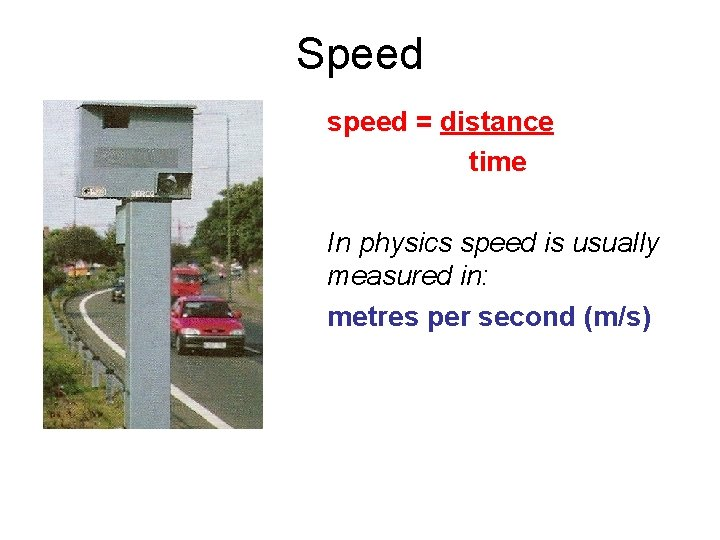Speed speed = distance time In physics speed is usually measured in: metres per second (m/s)also: distance = speed x time and: time = distance speed time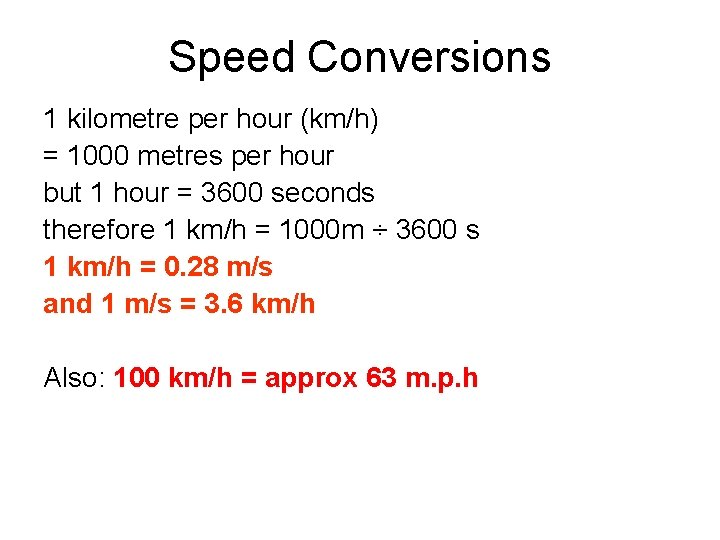Speed Conversions 1 kilometre per hour (km/h) = 1000 metres per hour but 1 hour = 3600 seconds therefore 1 km/h = 1000 m ÷ 3600 s 1 km/h = 0. 28 m/s and 1 m/s = 3. 6 km/h Also: 100 km/h = approx 63 m. p. h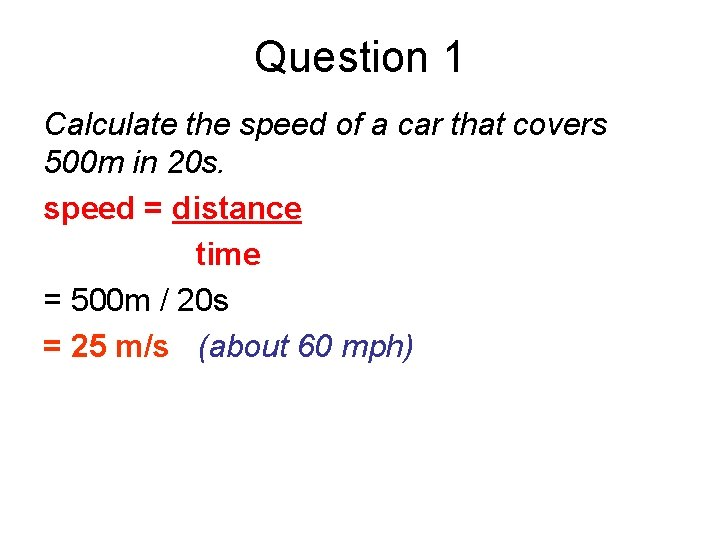Question 1 Calculate the speed of a car that covers 500 m in 20 s. speed = distance time = 500 m / 20 s = 25 m/s (about 60 mph)Question 2 Sound waves travel at about 340 m/s through air. How far will a sound wave travel in one minute? distance = speed x time = 340 m/s x 1 minute = 340 m/s x 60 seconds = 20 400 m (20. 4 km or about 13 miles)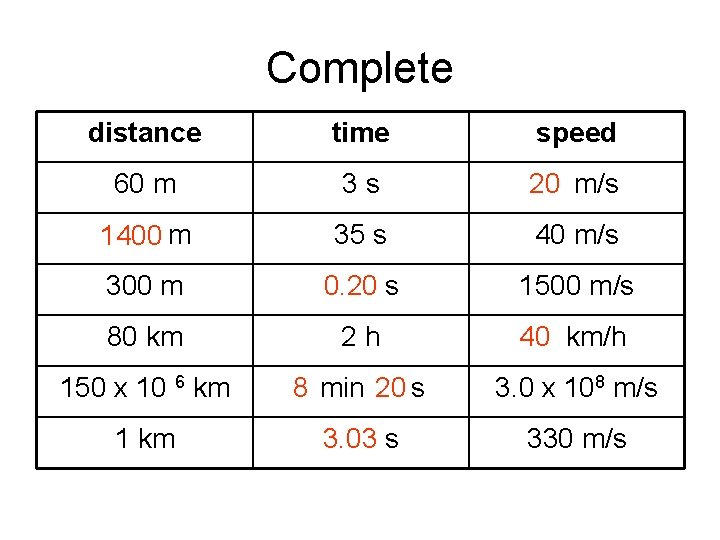Complete distance time speed 60 m 3 s 20 20 m/s 1400 m 35 s 40 m/s 300 m 0. 20 s 1500 m/s 80 km 2 h 40 km/h 40 150 x 10 6 km 88 min 20 s 3. 0 x 108 m/s 1 km 3. 03 s 330 m/sDistance-time graphs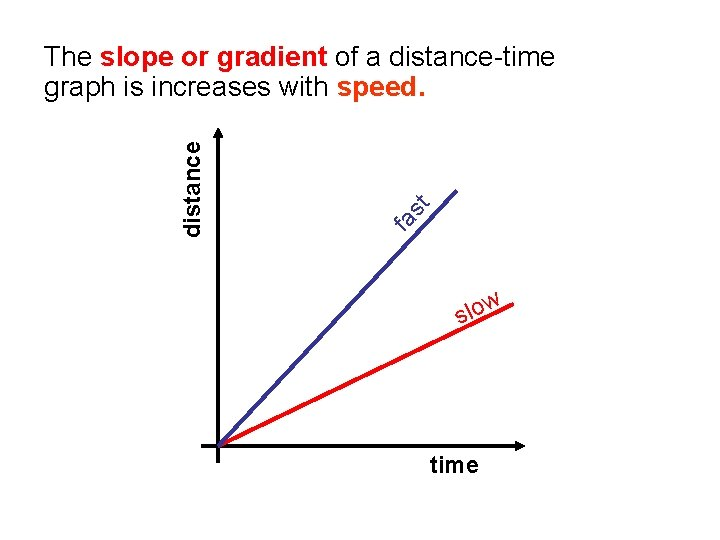st fa distance The slope or gradient of a distance-time graph is increases with speed. w o l s time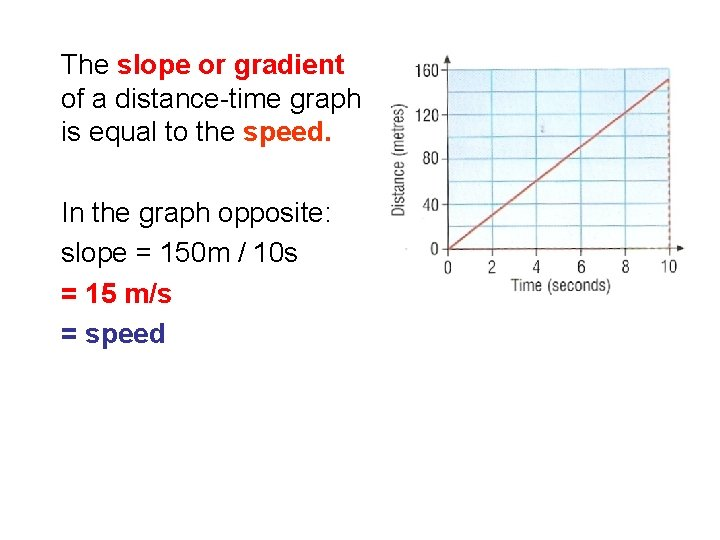The slope or gradient of a distance-time graph is equal to the speed. In the graph opposite: slope = 150 m / 10 s = 15 m/s = speedlorry bu s Sketch on the same set of axes distancetime graphs for: (a) a car moving at a steady speed, (b) a bus moving at a steady speed greater than the car, (c) a lorry increasing in speed from rest. distance Question 1 car time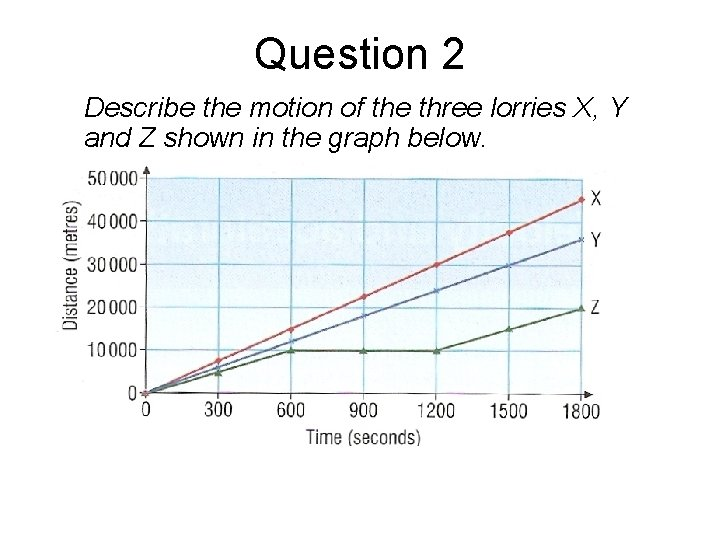Question 2 Describe the motion of the three lorries X, Y and Z shown in the graph below.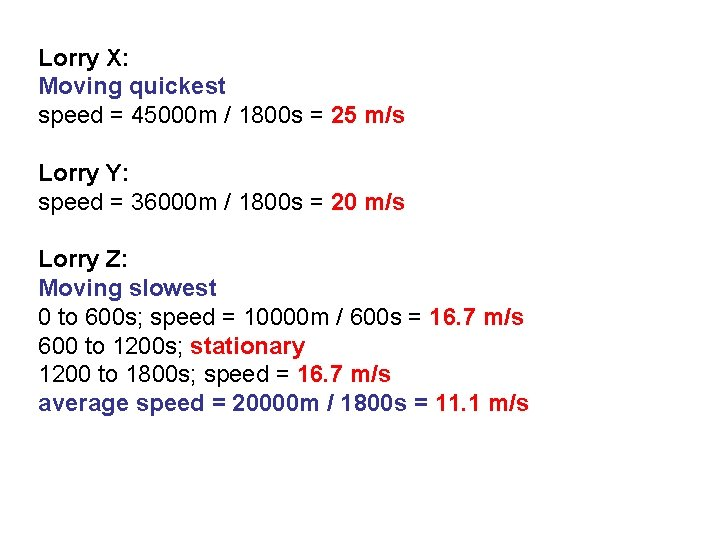Lorry X: Moving quickest speed = 45000 m / 1800 s = 25 m/s Lorry Y: speed = 36000 m / 1800 s = 20 m/s Lorry Z: Moving slowest 0 to 600 s; speed = 10000 m / 600 s = 16. 7 m/s 600 to 1200 s; stationary 1200 to 1800 s; speed = 16. 7 m/s average speed = 20000 m / 1800 s = 11. 1 m/sChoose appropriate words to fill in the gaps below: distance divided by time and can be Speed is equal to ____ metres measured in _____ per second. 72 A speed of 20 m/s is the same as ______ km/h which is 40 approximately equal to ______ mph. slope The _____ of a distance against time graph can be used to calculate ____. The greater the gradient of the line the speed higher is the speed. The line will be ______ horizontal _____ when the speed is zero. WORD SELECTION: slope 40 speed higher distance horizontal 72 metresVelocity The velocity of a body is its speed in a given direction. The airplane opposite may loop at a constant speed but its velocity changes as its direction of motion changes.Question A stone dropped off the top of a cliff falls down by 20 m in 2 s. Calculate its average velocity (a) downwards and (b) horizontally. (a) average speed downwards = 20 m / 2 s = 10 m/s Therefore velocity downwards = 10 m/s (b) average speed horizontally = 0 m / 2 s = 0 m/s Therefore velocity horizontally = 0 m/sAcceleration acceleration = velocity change time taken acceleration is measured in: metres per second squared (m/s 2)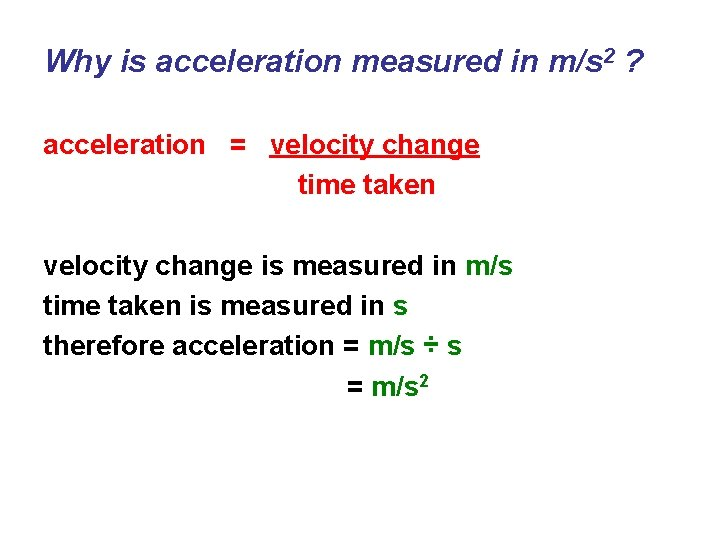Why is acceleration measured in m/s 2 ? acceleration = velocity change time taken velocity change is measured in m/s time taken is measured in s therefore acceleration = m/s ÷ s = m/s 2Other notes: 1. Speed and velocity: Often, but not always, speed can be used in the equation. 2. Change in velocity: = final velocity – initial velocity 3. Deceleration: This is where the speed is decreasing with time. 4. Circular motion at a constant speed: Acceleration is occurring because the direction of motion is continually changing and hence so is velocity.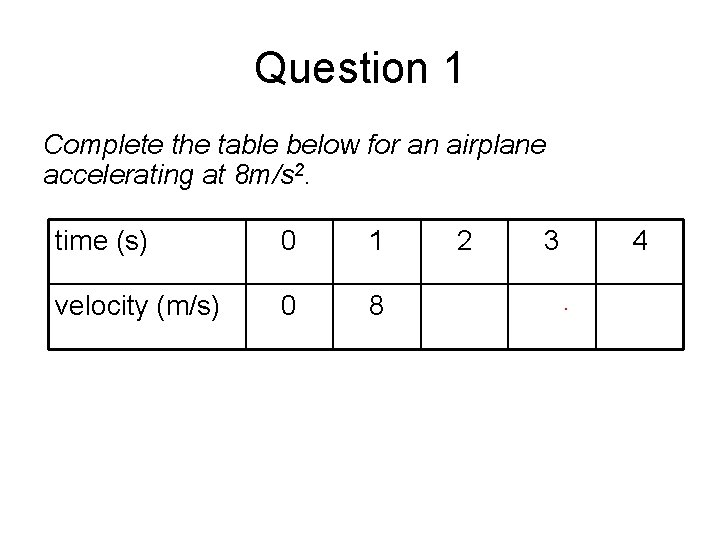Question 1 Complete the table below for an airplane accelerating at 8 m/s 2. time (s) 0 1 2 3 4 velocity (m/s) 0 8 16 24 32Question 2 Calculate the acceleration of a car that changes in velocity from 5 m/s to 25 m/s in 4 seconds. acceleration = velocity change time taken = (25 m/s – 5 m/s) / 4 s = 20 / 4 acceleration = 5 m/s 2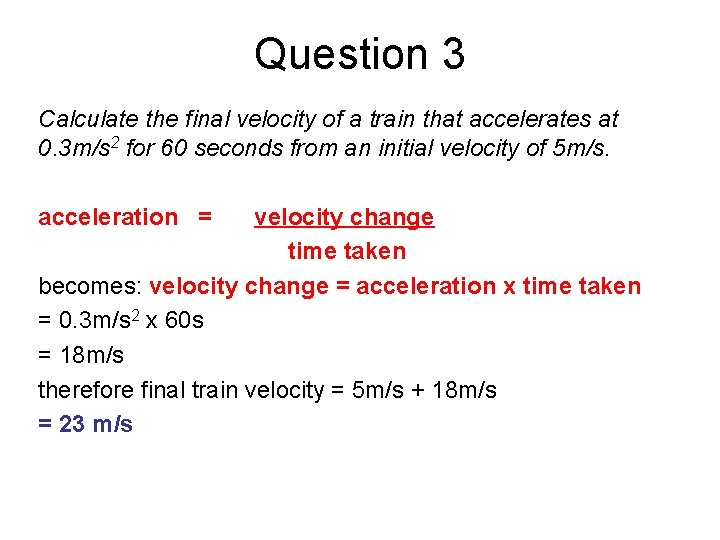Question 3 Calculate the final velocity of a train that accelerates at 0. 3 m/s 2 for 60 seconds from an initial velocity of 5 m/s. acceleration = velocity change time taken becomes: velocity change = acceleration x time taken = 0. 3 m/s 2 x 60 s = 18 m/s therefore final train velocity = 5 m/s + 18 m/s = 23 m/s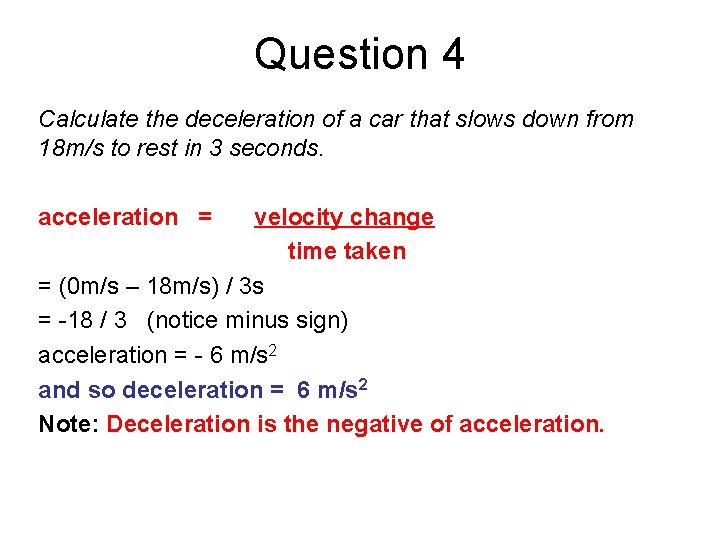Question 4 Calculate the deceleration of a car that slows down from 18 m/s to rest in 3 seconds. acceleration = velocity change time taken = (0 m/s – 18 m/s) / 3 s = -18 / 3 (notice minus sign) acceleration = - 6 m/s 2 and so deceleration = 6 m/s 2 Note: Deceleration is the negative of acceleration.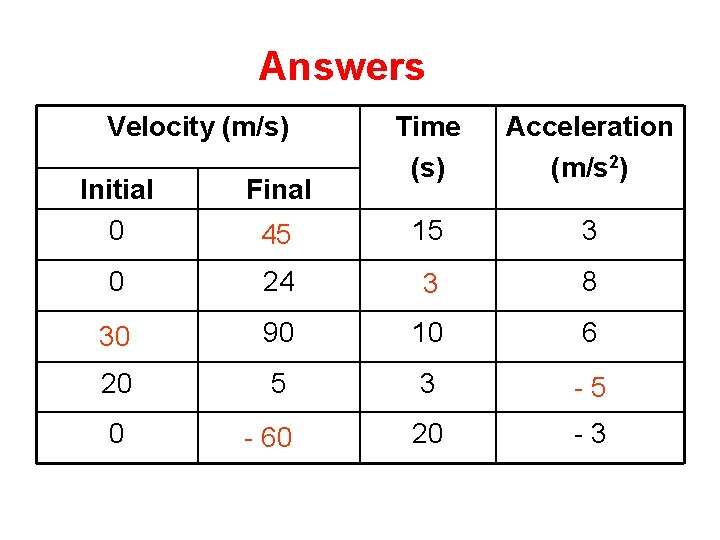Answers Complete Velocity (m/s) Time (s) Acceleration (m/s 2) 15 3 Initial 0 Final 45 0 24 3 8 30 90 10 6 20 5 3 -5 0 60 - -60 20 -3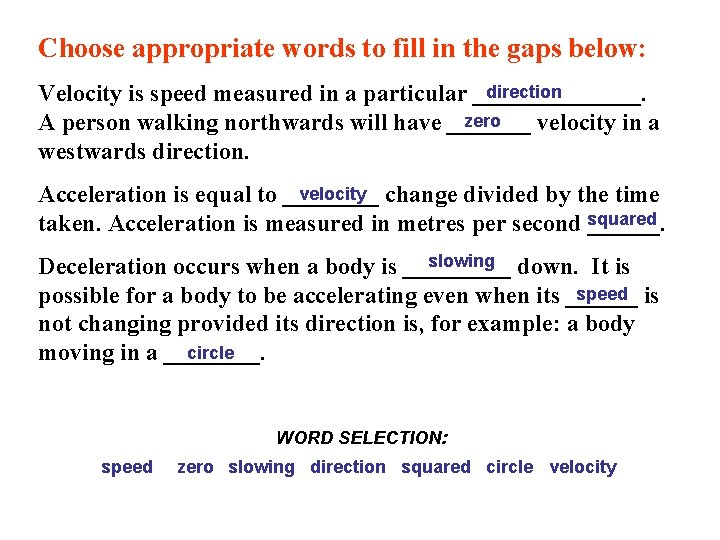Choose appropriate words to fill in the gaps below: direction Velocity is speed measured in a particular _______. zero A person walking northwards will have _______ velocity in a westwards direction. velocity change divided by the time Acceleration is equal to ____ taken. Acceleration is measured in metres per second squared ______. slowing down. It is Deceleration occurs when a body is _____ speed is possible for a body to be accelerating even when its ______ not changing provided its direction is, for example: a body circle moving in a ____. WORD SELECTION: speed zero slowing direction squared circle velocity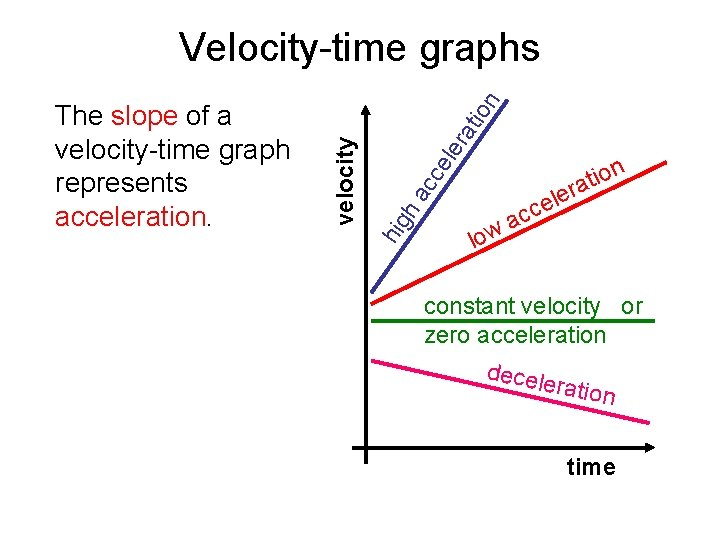ra ele cc ha hig velocity The slope of a velocity-time graph represents acceleration. tio n Velocity-time graphs low le e c ac n o i t ra constant velocity or zero acceleration decele ration timevelocity The area under a velocity-time graph represents distance travelled. area equals distance travelled timeQuestion 1 Sketch the velocity time graph of a car accelerating from rest to 15 m/s in 3 seconds and then remaining at a constant speed for one more second. velocity (m/s) 15 10 5 1 2 3 4 time (s)Question 2 Calculate the acceleration and the distance travelled after 4 seconds from using the graph opposite. acceleration = gradient = y-step ÷ x-step = (12 - 0)m/s ÷ (4 – 0)s = 12 / 4 acceleration = 3 m/s 2 distance = area under the graph = area of triangle = ½ x base x height = ½ x 4 s x 12 m/s distance travelled = 24 m velocity (m/s) 12 8 4 area 1 2 3 4 time (s)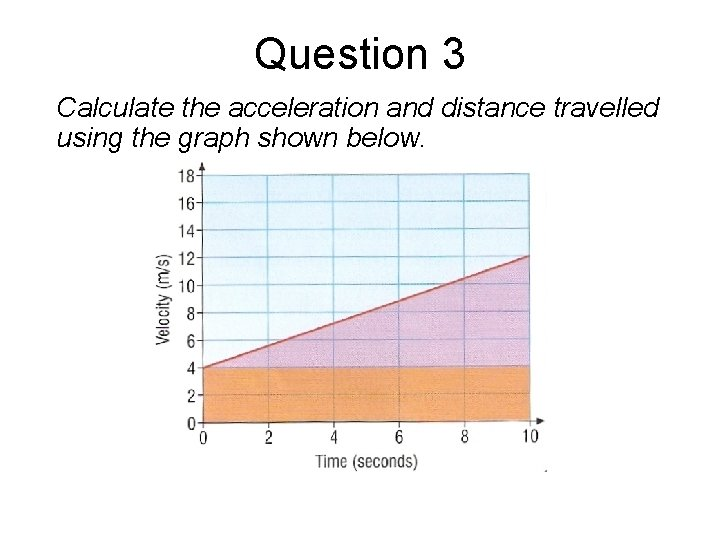Question 3 Calculate the acceleration and distance travelled using the graph shown below.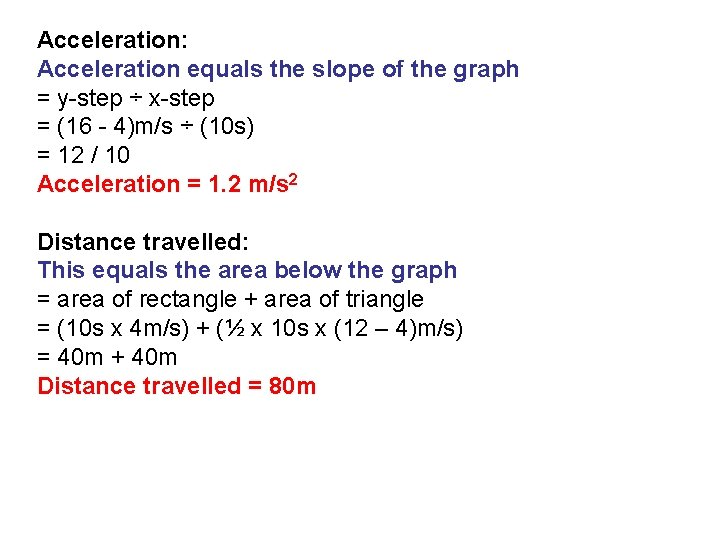Acceleration: Acceleration equals the slope of the graph = y-step ÷ x-step = (16 - 4)m/s ÷ (10 s) = 12 / 10 Acceleration = 1. 2 m/s 2 Distance travelled: This equals the area below the graph = area of rectangle + area of triangle = (10 s x 4 m/s) + (½ x 10 s x (12 – 4)m/s) = 40 m + 40 m Distance travelled = 80 mQuestion 4 Calculate the distance travelled over 15 seconds and the deceleration during the final five seconds using the graph below.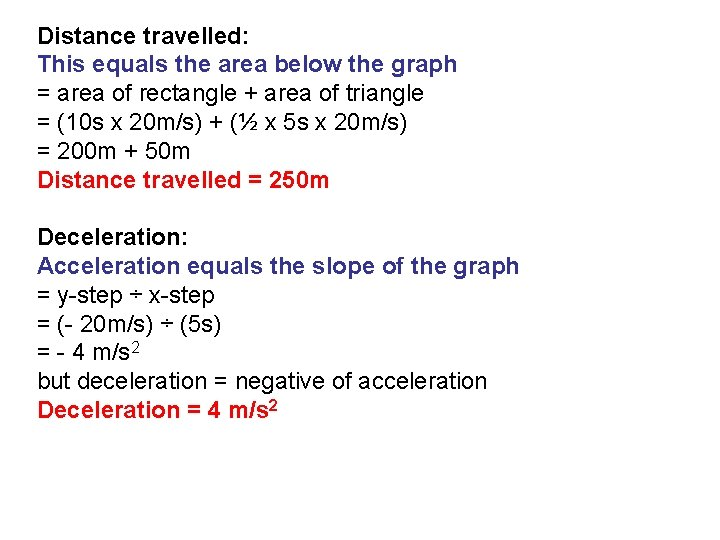Distance travelled: This equals the area below the graph = area of rectangle + area of triangle = (10 s x 20 m/s) + (½ x 5 s x 20 m/s) = 200 m + 50 m Distance travelled = 250 m Deceleration: Acceleration equals the slope of the graph = y-step ÷ x-step = (- 20 m/s) ÷ (5 s) = - 4 m/s 2 but deceleration = negative of acceleration Deceleration = 4 m/s 2Online Simulations The Moving Man - Ph. ET - Learn about position, velocity, and acceleration graphs. Move the little man back and forth with the mouse and plot his motion. Set the position, velocity, or acceleration and let the simulation move the man for you. Maze Game - Ph. ET - Learn about position, velocity, and acceleration in the "Arena of Pain". Use the green arrow to move the ball. Add more walls to the arena to make the game more difficult. Try to make a goal as fast as you can. Motion in 2 D - Ph. ET - Learn about velocity and acceleration vectors. Move the ball with the mouse or let the simulation move the ball in four types of motion (2 types of linear, simple harmonic, circle). See the velocity and acceleration vectors change as the ball moves. Ladybug motion in 2 D - Ph. ET - Learn about position, velocity and acceleration vectors. Move the ladybug by setting the position, velocity or acceleration, and see how the vectors change. Choose linear, circular or elliptical motion, and record and playback the motion to analyze the behavior Motion with constant acceleration - Fendt Bouncing ball with motion graphs - netfirms Displacement-time graph with set velocities NTNU Displacement & Aceleration-time graphs with set velocities - NTNU Displacement & Velocity-time graphs with set accelerations - NTNU Football distance-time graphs - e. Chalk Motion graphs with tiger - NTNU Two dogs running with graphs - NTNU Motion graphs test - NTNU BBC AQA GCSE Bitesize Revision: Speed, distance and time Distance-time graphs Velocity-time graphs Acceleration Distance-time graphs (higher) Velocity-time graphs (higher)